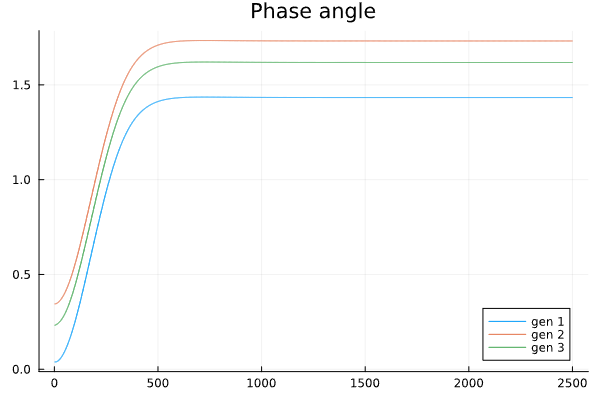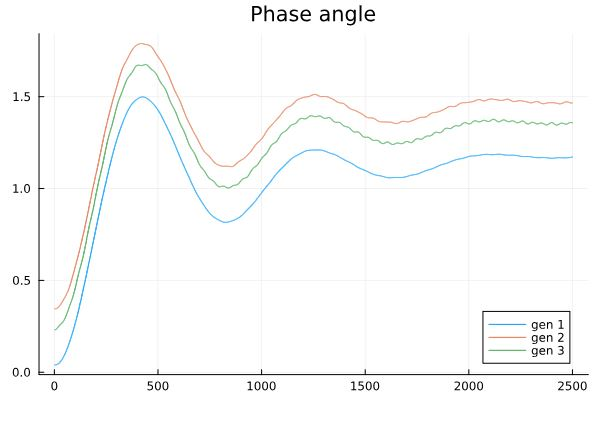# Modified the neural network to capture nonlinear system

I tried to use NeuralPDE to solve ODE problem and my code is below:

``````domains = [t ∈ Interval(0.0,25.0)]

chain =[Lux.Chain(Dense(1,10,Lux.tanh),Dense(10,20,Lux.tanh),Dense(20,10,Lux.tanh),Dense(10,1)) for _ in 1:12]

dvs = [delta1(t),delta2(t),delta3(t),omega1(t),omega2(t),omega3(t),TM1(t),TM2(t),TM3(t),Psv1(t),Psv2(t),Psv3(t)]

@named pde_system = PDESystem(eqs,bcs,domains,[t],dvs)

strategy = NeuralPDE.GridTraining(0.01)
sym_prob = NeuralPDE.symbolic_discretize(pde_system, discretization)

pde_loss_functions = sym_prob.loss_functions.pde_loss_functions
bc_loss_functions = sym_prob.loss_functions.bc_loss_functions

callback = function (p, l)
println("loss: ", l)
return false
end
loss_functions =  [pde_loss_functions;bc_loss_functions]

function loss_function(θ,p)
sum(map(l->l(θ) ,loss_functions))
end

f_ = OptimizationFunction(loss_function, Optimization.AutoZygote())
prob = Optimization.OptimizationProblem(f_, sym_prob.flat_init_params);
phi = sym_prob.phi;
``````

I got the result for the phase angle from the NeuralPDE and the classical method as follows:
NeuralPDEClassical methodWhen I compared the result from NeuralPDE with the results from the classical method I think it was good for the first 5 seconds. But the NeuralPDE can not capture the problem after 5 seconds, although I have set the time domains is 25 seconds. (I also try to set the domains longer but got the same results).
Please help me with how could I modify the NeuralPDE to expand the network to hold time domains.
Thank you all.

Can you open an issue? This would be a good test case for something like adaptive reweighing.

I am sorry, I do not know what you mean. You asked me that have I solved it or do you want me to open the issue? (make as having solution)

I want you to open an issue on NeuralPDE.jl so someone can take a deeper look.

I have created a new issue on NeuralPDE.jl GitHub.